# Class 11 Maths NCERT Solutions for Chapter 7 Permutations and Combinations Exercise 7.2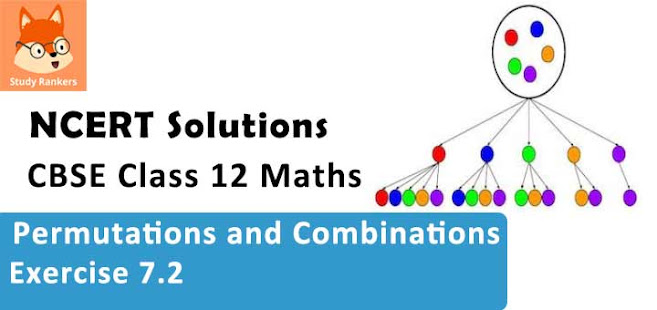### Permutations and Combinations Exercise 7.2 Solutions

1. Evaluate
(i) 8!
(ii) 4! – 3!

Solution

(i) 8! = 1 × 2 × 3 × 4 × 5 × 6 × 7 × 8 = 40320
(ii) 4! = 1 × 2 × 3 × 4 = 24
3! = 1 × 2 × 3 = 6
∴ 4! – 3! = 24 – 6 = 18

2. Is 3! + 4! = 7!?

Solution

3! = 1 × 2 × 3 = 6
4! = 1 × 2 × 3 × 4 = 24
∴3! + 4! = 6 + 24 = 30
7! = 1 × 2 × 3 × 4 × 5 × 6 × 7 = 5040
∴ 3! + 4! ≠ 7!

3.Compute 8!/(6! × 2!)

Solution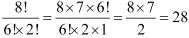4. If 1/6! + 1/7! = x/8! , find x.
Solution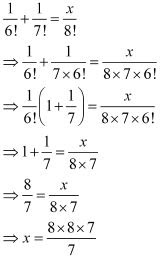∴ x = 64

5 Evaluate n!/(n - r)! , when
(i) n = 6, r = 2
(ii) n = 9, r = 5
Solution
(i)  When n = 6, r = 2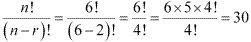(ii) When n = 9, r = 5,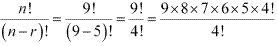= 9 × 8 ×7 × 6 × 5 = 15120